# House Sales in King County¶

In this project, I am analyzing a dataset of about 21,000 house sales in King County (Seattle) over the course of a year (in 2014/15). The data are taken from a Kaggle competition. The goal is to build a model predicting sales prices.

In the first section, I will explore the correlation between the different features and the target variable (price) and visualize the data. In the second part, I will build a predictive regression model. In order to do so, I will use different regression algorithms and various different feature selection and engineering techniques. The best model is linear regression with data preprocessed by creating quadratic polynomial features. This model has an accuracy of 0.82 (R2 score).

In :
from IPython.core.display import display, HTML
display(HTML("<style>.container { width:60% !important; }</style>"))

In :
import numpy as np
import pandas as pd
import matplotlib.pyplot as plt
import seaborn as sns

from sklearn.linear_model import LinearRegression
from sklearn.model_selection import cross_val_score
from sklearn.pipeline import make_pipeline
from sklearn.preprocessing import PolynomialFeatures
from sklearn.model_selection import train_test_split
from sklearn import linear_model
from sklearn.preprocessing import StandardScaler
from sklearn.feature_selection import RFE
from sklearn.model_selection import validation_curve
from sklearn.svm import SVR
from sklearn.ensemble import RandomForestRegressor
from sklearn.pipeline import Pipeline
from sklearn.decomposition import PCA


First, the dataset is loaded from a csv file and the date column is converted from string to a timestamp.

In :
df = pd.read_csv('kc_house_data.csv')

In :
# Convert date to timestamp
df['date'] = pd.to_datetime(df['date'])

In :
df.head()

Out:
id date price bedrooms bathrooms sqft_living sqft_lot floors waterfront view ... grade sqft_above sqft_basement yr_built yr_renovated zipcode lat long sqft_living15 sqft_lot15
0 7129300520 2014-10-13 221900.0 3 1.00 1180 5650 1.0 0 0 ... 7 1180 0 1955 0 98178 47.5112 -122.257 1340 5650
1 6414100192 2014-12-09 538000.0 3 2.25 2570 7242 2.0 0 0 ... 7 2170 400 1951 1991 98125 47.7210 -122.319 1690 7639
2 5631500400 2015-02-25 180000.0 2 1.00 770 10000 1.0 0 0 ... 6 770 0 1933 0 98028 47.7379 -122.233 2720 8062
3 2487200875 2014-12-09 604000.0 4 3.00 1960 5000 1.0 0 0 ... 7 1050 910 1965 0 98136 47.5208 -122.393 1360 5000
4 1954400510 2015-02-18 510000.0 3 2.00 1680 8080 1.0 0 0 ... 8 1680 0 1987 0 98074 47.6168 -122.045 1800 7503

5 rows × 21 columns

# 1. Exploratory Data Analysis¶

First, I'm going to gather some basic information about the data set:

In :
df.shape

Out:
(21613, 21)
In :
df.columns

Out:
Index(['id', 'date', 'price', 'bedrooms', 'bathrooms', 'sqft_living',
'sqft_lot', 'floors', 'waterfront', 'view', 'condition', 'grade',
'sqft_above', 'sqft_basement', 'yr_built', 'yr_renovated', 'zipcode',
'lat', 'long', 'sqft_living15', 'sqft_lot15'],
dtype='object')

There are 21,613 house sales in this data set, each of which has 21 features (listed above).

In :
df.dtypes

Out:
id                        int64
date             datetime64[ns]
price                   float64
bedrooms                  int64
bathrooms               float64
sqft_living               int64
sqft_lot                  int64
floors                  float64
waterfront                int64
view                      int64
condition                 int64
sqft_above                int64
sqft_basement             int64
yr_built                  int64
yr_renovated              int64
zipcode                   int64
lat                     float64
long                    float64
sqft_living15             int64
sqft_lot15                int64
dtype: object

All of the features are numerical, except for the date of the transaction.

## 1.1. Correlation Matrix¶

In :
df.corr()['price'].sort_values(ascending=False)

Out:
price            1.000000
sqft_living      0.702035
sqft_above       0.605567
sqft_living15    0.585379
bathrooms        0.525138
view             0.397293
sqft_basement    0.323816
bedrooms         0.308350
lat              0.307003
waterfront       0.266369
floors           0.256794
yr_renovated     0.126434
sqft_lot         0.089661
sqft_lot15       0.082447
yr_built         0.054012
condition        0.036362
long             0.021626
id              -0.016762
zipcode         -0.053203
Name: price, dtype: float64

Looking at the correlation between price and the other features, one can notice that the strongest correlation is between price and sqft_living (0.7), grade (0.67), and sqft_above (0.61). Not surprisingly, there is virtually no correlation between price and zipcode, id, and longitude.

The following correlation matrix shows the correlation between any two features of the data set.

In :
plt.figure(figsize=(12,12))
sns.heatmap(df.corr(),vmax=1.0,vmin=-1.0, square=True, fmt='.2f',
annot=True, cbar_kws={"shrink": .75}, cmap='coolwarm')
plt.show()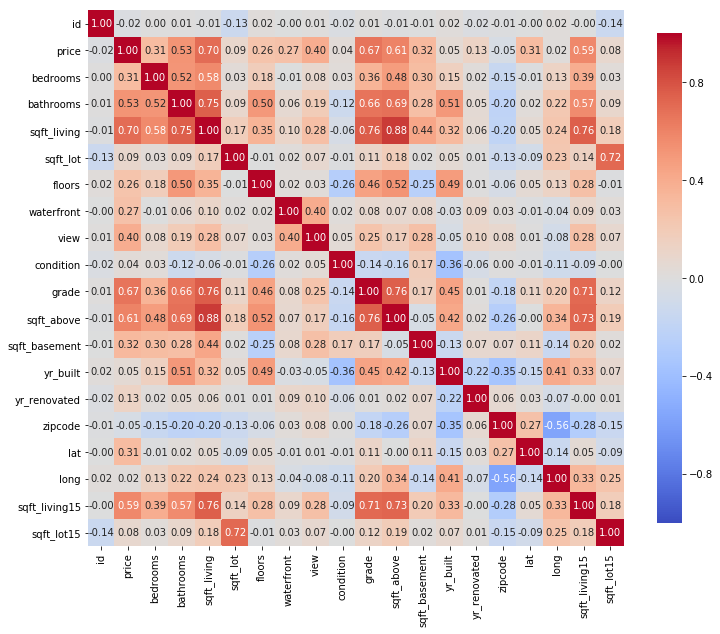In addition to the correlations between price and other features, there are some other noteworthy facts about the correlation matrix: the strongest correlation is between sqft_living and sqft_above, the srongest negative correlation is between zipcode and longitode, suggesting that zipcodes in King County were drawn from east to west. Generally, there is a strong correlation between the quantities that relate to the size of the house: sqft_living, sqft_living15, sqft_above, bedrooms, and bathrooms.

# 1.2. House Prices¶

Below is a histogram of the sales prices. Most prices are between \$200,000 and \$1,000,000.

In :
plt.hist(df['price'], bins=200, alpha=0.3)
plt.grid()
plt.xlabel('House price ($)') plt.ylabel('Transactions') plt.xlim(0,1200000) plt.show()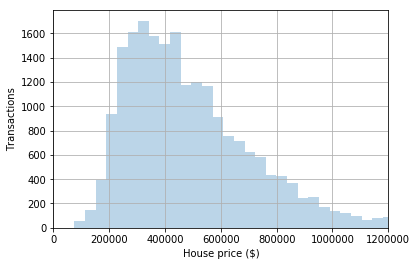In : print('Maximum house price: \t${:0,.2f}'.format(df['price'].max()))
print('Minimum house price: \t${:0,.2f}'.format(df['price'].min())) print('Mean house price: \t${:0,.2f}'.format(df['price'].mean()))
print('Median house price: \t${:0,.2f}'.format(df['price'].median()))  Maximum house price:$7,700,000.00
Minimum house price: 	$75,000.00 Mean house price:$540,088.14
Median house price: 	$450,000.00  Next, I will group the data by date and then day of the week to get a sense for how the number of transactions varies over the course of a year and a week. The a significant dips in transactions in the winter and on the weekend. In : # Group transactions by date df_date = df.groupby('date', as_index=False).count() # Plot transactions throughout a year plt.figure(figsize=(12,6)) plt.plot(df_date['date'], df_date['id']) plt.xlabel('Date') plt.ylabel('Transactions') plt.grid() plt.show()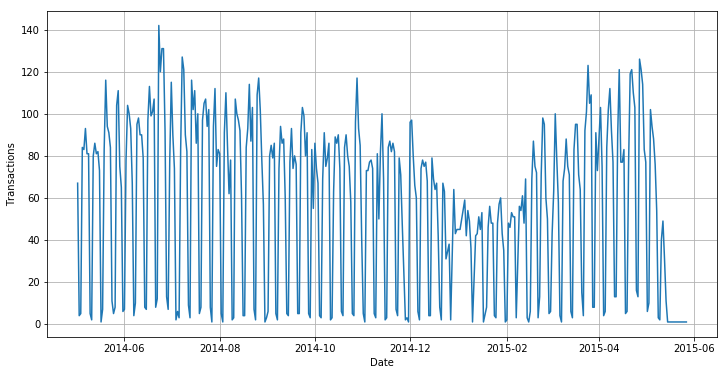In : df_week = df.copy() df_week['day_of_week'] = df_week['date'].dt.dayofweek df_week = df_week.groupby('day_of_week', as_index=False).count()  In : plt.bar(df_week['day_of_week'], df_week['id']) plt.xlabel('Day of Week') plt.ylabel('Transactions') plt.xticks(np.arange(7), ('Mon', 'Tue', 'Wed', 'Thu', 'Fri','Sat','Sun')) plt.show()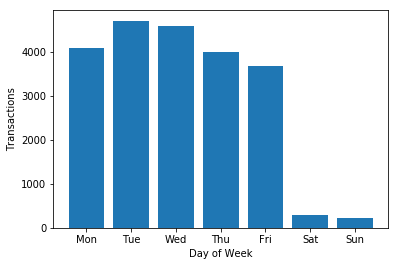The feature most correlated with price is the size of the living area sqft_living. Here, I'm visualizing the relationship of these two quantities in a hexagonal binning plot. In : plt.hexbin(df['sqft_living'], df['price'], gridsize=150, cmap='Blues') plt.xlim(0,4000) plt.ylim(0,1500000) plt.xlabel('Square Footage of Living Space') plt.ylabel('Price (USD)') plt.show()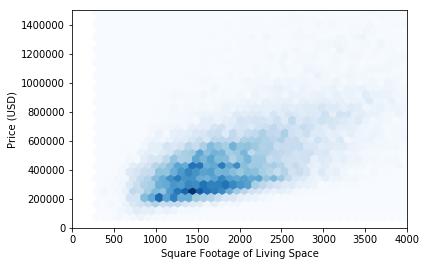Some ids appear more than once in the dataset, indicating that the house was sold twice within a year. By grouping the data by id and filtering the original data, I can investigate these properties. For example, the below histogram shows their sales price distribution. In : # Houses sold more than once df_id = df.groupby('id', as_index=False).count()[['id','date']] df_id = df_id[df_id['date']>1][['id']] df_twice = df_id.merge(df, on='id', how='inner') df_twice['price'].hist(bins=50) plt.xticks(rotation=60) plt.xlabel('Price (USD)') plt.ylabel('Transactions') plt.show()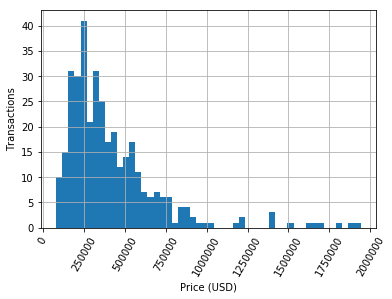One example is the house with id 7200179 which was sold in October 2014 for \$150,000 and then sold again for \$175,000 six months later. In : df_twice[df_twice['id']==7200179]  Out: id date price bedrooms bathrooms sqft_living sqft_lot floors waterfront view ... grade sqft_above sqft_basement yr_built yr_renovated zipcode lat long sqft_living15 sqft_lot15 2 7200179 2014-10-16 150000.0 2 1.0 840 12750 1.0 0 0 ... 6 840 0 1925 0 98055 47.484 -122.211 1480 6969 3 7200179 2015-04-24 175000.0 2 1.0 840 12750 1.0 0 0 ... 6 840 0 1925 0 98055 47.484 -122.211 1480 6969 2 rows × 21 columns # 2. Pridictive Model for House Prices¶ In the following, I will build a predictive regression model which will predic the price of a house based on the various features of the house. In order to prepare the data set for model building, the date column is transformed into numerical features (year, month, day, and day of the week) and the data are split into a training and a test set. In addition, a standardized feature matrix X_std is created. In : # Split data into features and target X = df.drop('price', axis=1) y = df['price'] # Transform date from single timestamp feature to multiple numerical # features X['date_day'] = X['date'].dt.day X['date_month'] = X['date'].dt.month X['date_year'] = X['date'].dt.year X['date_DoW'] = X['date'].dt.dayofweek X = X.drop('date', axis=1) # Split data set into training and test sets X_train, X_test, y_train, y_test = \ train_test_split(X, y, test_size=0.2, random_state=0) # Create standardized feature matrix X_std = StandardScaler().fit_transform(X_train)  ## 2.1. Simple Model: Linear Regression¶ The simplest approach to this regression task is an ordinary least squares regression. I'm using the LinearRegression regressor implemented in scikit-learn. The performance of the regressor is evaluated by 10-fold cross-validation on the training set using the R2 score as the scoring metric. In : # Linear Regression lr = LinearRegression() # Evaluate model with cross-validation cvs = cross_val_score(estimator=lr, X=X_train, y=y_train, cv=10, scoring='r2') print('CV score: %.3f ± %.3f' % (cvs.mean(), cvs.std()))  CV score: 0.699 ± 0.017  This simple model achieves an R2 score of 0.70 and we can use the coefficients of the individual features to gain quantiative insights into what affects the pricing of the houses. In : lr.fit(X_train, y_train) coef_list = list(lr.coef_) name_list = list(X_train.columns) pd.Series(coef_list, index=name_list)  Out: id -0.000001 bedrooms -34997.381606 bathrooms 39528.155881 sqft_living 112.819757 sqft_lot 0.166812 floors 8015.002050 waterfront 606251.458750 view 52595.525562 condition 27551.746953 grade 93888.181170 sqft_above 72.775118 sqft_basement 40.044639 yr_built -2604.681249 yr_renovated 21.102001 zipcode -569.772923 lat 606869.941131 long -220289.504081 sqft_living15 24.177352 sqft_lot15 -0.478231 date_day -369.592046 date_month 1359.265288 date_year 38013.762706 date_DoW 465.707124 dtype: float64 According to this model, every square foot of living space adds \$112 to the property value, every bathroom adds \$39,528, and a waterfron view adds \$606,251. While these numbers may sound reasonable, the is cause for scepticism looking at some of the other predictions: it seems counter-intuitive that each bedroom would *reduce* the value by \\$34,997 and that the size of the lot has no influence on the pricing.

Since I will be evaluating various regressors in combination with other estimators, the following function is defined as a shortcut to evaluate a model:

In :
def eval_model(estimator, X=X_train, y=y_train, out=True):
'''
Evaluates a model provided in 'estimator' and returns the
cross-validation score (as a list of 10 results)
X: Feature matrix
y: Targets
'''
cvs = cross_val_score(estimator=estimator, X=X, y=y,
cv=10, scoring='r2')

if out == True:
print('CV score: %.3f ± %.3f' % (cvs.mean(), cvs.std()))

return cvs


## 2.2. Feature Selection¶

In this section I will try to reduce the number of features in order to possibly reduce the effects of overfitting and reduce training time. First, I will build a model with successively more relevant features, second, I will use recursive feature elimination (RFE).

### 2.2.1. Add features according to their correlation with price¶

The first idea is to start building a very simple model with only one feature, namely the one with the highest correlation with price, namely sqft_living. Then, more features are added in order of their correlation with price. For each model, a score is calculated.

The following code segment produces a Series of features and their respective correlations with price.

In :
# Calculate correlation or each feature and create sorted
# pandas Series of correlation factors
corr_ranking = df.corr()['price'].sort_values(ascending=False)[1:]
corr_ranking

Out:
sqft_living      0.702035
sqft_above       0.605567
sqft_living15    0.585379
bathrooms        0.525138
view             0.397293
sqft_basement    0.323816
bedrooms         0.308350
lat              0.307003
waterfront       0.266369
floors           0.256794
yr_renovated     0.126434
sqft_lot         0.089661
sqft_lot15       0.082447
yr_built         0.054012
condition        0.036362
long             0.021626
id              -0.016762
zipcode         -0.053203
Name: price, dtype: float64

Now, I will loop over the features sorted by correlation and successively add more and more features to the model. At each step, the CV score is calculated and stored in score_list. The order in which features were added is stored in feat_list.

In :
feat_list = []       # List of features added to the model
score_list = []      # List of CV scores obtained after adding features

# Loop over features in order of correlation with price
for feat in list(corr_ranking.index):

feat_list.append(feat)      # Add feature name to feat_list

# Calculate CV score
cvs = eval_model(lr, X=X_train[feat_list], out=False)

score_list.append(cvs.mean())   # Add score to score_list


The following plot shows the improvement of the model with each new feature added. sqft_living along yields a score of 0.49 and the score continuously increases with every feature. There are only a few features that do not inprove the model and none that reduce its score. The contributions of the individual features is indicated by the orange bars.

In :
fig, ax = plt.subplots(1,1, figsize=(12,6))

# Accumulated CV score
ax.plot(score_list)
ax.set_xticks(list(range(len(feat_list))))
ax.set_xticklabels(feat_list, rotation=60)
ax.set_ylabel('CV score (R2) (blue)')
ax.grid()

# Derivative
axR = ax.twinx()
axR.set_ylabel('Individual feature contribution (orange)')
axR.bar(left=list(range(19)), height=np.diff(+score_list),
alpha=0.4, color='orange')

plt.show()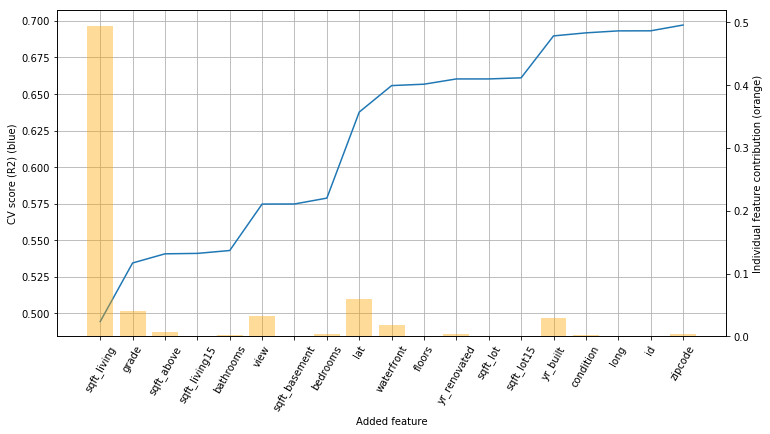### 2.2.2. Recursive Feature Elimination (RFE)¶

Next, I will use scikit-learn's RFE implementation to eliminate features in a more sophisticated manner. In the following, RFE is performed for any an increasing number of features to be selected n and for each model, the score is recorded in scores_list. The below plot shows how the score increases are n increases.

In :
features_range = range(1,24)
scores_list = []

for n in features_range:
rfe = RFE(lr, n, step=1)
rfe.fit(X_train, y_train)
scores_list.append(rfe.score(X_train, y_train))

In :
fig, ax = plt.subplots(1,1, figsize=(12,6))

# Regular sized plot
ax.plot(features_range, scores_list)
ax.set_xlabel('Number of features')
ax.set_ylabel('CV score (R2)')
ax.set_xticks(features_range)
ax.grid()

# Vertical magnification
axR = ax.twinx()
axR.plot(features_range, scores_list/max(scores_list), linestyle='--')
axR.set_ylabel('% of maximum CV score (dashed)')
axR.set_ylim(0.94,1.01)

plt.show()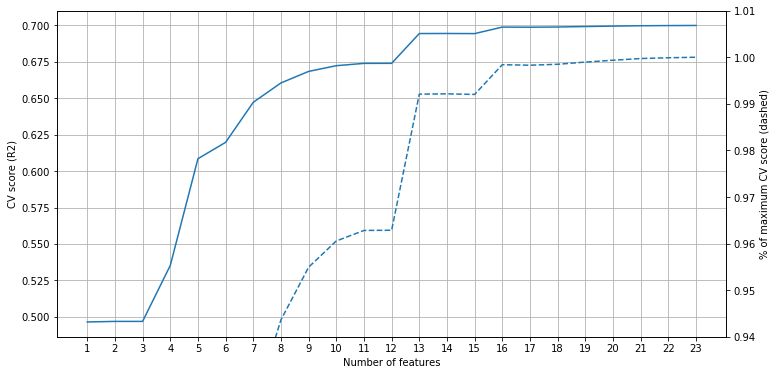The model's score approaches the maximum value relatively quickly and already after 13 features, the score is above 99% of the final score, meaning that 10 features (almost half) can be discarded without significantly impacting the model accuracy. These are those 13 features:

In :
# Fit RFE model with n_features_to_select=13 to X_train
rfe = RFE(lr, 13, step=1)
rfe.fit(X_train, y_train)

# Print list of selected columns
list(X_train.columns[rfe.support_])

Out:
['bedrooms',
'bathrooms',
'sqft_living',
'waterfront',
'view',
'condition',
'sqft_above',
'sqft_basement',
'yr_built',
'lat',
'long',
'date_year']

I'm creating a new feature matrix X_rfe containing only the 13 most relevant features.

In :
X_rfe = X_train[list(X_train.columns[rfe.support_])]


## 2.3. Different Regression Algorithms¶

So far I've only used ordiniary least squares regression, lr. The highest score achieved with this model was 0.70.

In :
eval_model(lr, X=X_std);

CV score: 0.699 ± 0.017


### 2.3.1. Ridge¶

Ridge regression is an alternative to ordinary least squares regression, the main difference being that large coefficients are penalized in this model. The strength of this regularization is determined by the hyperparameter alpha. With the default of alpha=1.0, ridge regression yields the same R2 score:

In :
ridge = linear_model.Ridge()
eval_model(ridge, X=X_std);

CV score: 0.699 ± 0.017


In the following, a validation curve is used to determine whether other parameters of alpha yield improved scores. As the plot below shows, this is not the case. In fact, the model score seems independent of alpha, except for values exceeding 1,000 for which the model quickly deteriorates.

In :
# Trying different alpha parameters for Ridge regression

test_int = np.logspace(-10, 6, 17)

train_scores, valid_scores = \
validation_curve(ridge, X_std, y_train, 'alpha',
test_int, cv=5)

# Plot validation curve
plt.figure(figsize=(12,6))
plt.title('Validation curve')
plt.semilogx(test_int, np.mean(train_scores, axis=1),
marker='o', label='Training data')
plt.semilogx(test_int, np.mean(valid_scores, axis=1),
marker='o', label='Validation data')
plt.legend()
plt.ylabel('CV score (R2)')
plt.xlabel('alpha parameter')
plt.grid()
plt.show()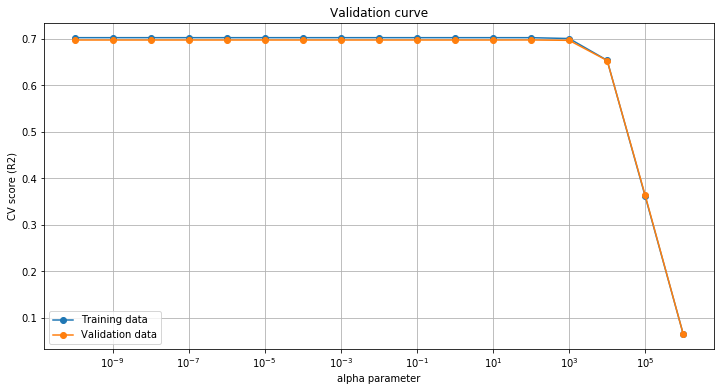### 2.3.2. Lasso¶

The same behavior (and no improvement in the R2 score) is observed for regression with lasso (L1) regularization. This is likely due to the relatively small number of features.

In :
lasso = linear_model.Lasso()
eval_model(lasso, X=X_std);

/usr/local/lib/python3.6/site-packages/sklearn/linear_model/coordinate_descent.py:491: ConvergenceWarning: Objective did not converge. You might want to increase the number of iterations. Fitting data with very small alpha may cause precision problems.
ConvergenceWarning)
/usr/local/lib/python3.6/site-packages/sklearn/linear_model/coordinate_descent.py:491: ConvergenceWarning: Objective did not converge. You might want to increase the number of iterations. Fitting data with very small alpha may cause precision problems.
ConvergenceWarning)
/usr/local/lib/python3.6/site-packages/sklearn/linear_model/coordinate_descent.py:491: ConvergenceWarning: Objective did not converge. You might want to increase the number of iterations. Fitting data with very small alpha may cause precision problems.
ConvergenceWarning)
/usr/local/lib/python3.6/site-packages/sklearn/linear_model/coordinate_descent.py:491: ConvergenceWarning: Objective did not converge. You might want to increase the number of iterations. Fitting data with very small alpha may cause precision problems.
ConvergenceWarning)
/usr/local/lib/python3.6/site-packages/sklearn/linear_model/coordinate_descent.py:491: ConvergenceWarning: Objective did not converge. You might want to increase the number of iterations. Fitting data with very small alpha may cause precision problems.
ConvergenceWarning)
/usr/local/lib/python3.6/site-packages/sklearn/linear_model/coordinate_descent.py:491: ConvergenceWarning: Objective did not converge. You might want to increase the number of iterations. Fitting data with very small alpha may cause precision problems.
ConvergenceWarning)
/usr/local/lib/python3.6/site-packages/sklearn/linear_model/coordinate_descent.py:491: ConvergenceWarning: Objective did not converge. You might want to increase the number of iterations. Fitting data with very small alpha may cause precision problems.
ConvergenceWarning)
/usr/local/lib/python3.6/site-packages/sklearn/linear_model/coordinate_descent.py:491: ConvergenceWarning: Objective did not converge. You might want to increase the number of iterations. Fitting data with very small alpha may cause precision problems.
ConvergenceWarning)
/usr/local/lib/python3.6/site-packages/sklearn/linear_model/coordinate_descent.py:491: ConvergenceWarning: Objective did not converge. You might want to increase the number of iterations. Fitting data with very small alpha may cause precision problems.
ConvergenceWarning)

CV score: 0.699 ± 0.017

/usr/local/lib/python3.6/site-packages/sklearn/linear_model/coordinate_descent.py:491: ConvergenceWarning: Objective did not converge. You might want to increase the number of iterations. Fitting data with very small alpha may cause precision problems.
ConvergenceWarning)

In :
# Trying different alpha parameters for Lasso regression

test_int = np.logspace(-10, 6, 17)

train_scores, valid_scores = \
validation_curve(lasso, X_std, y_train, 'alpha',
test_int, cv=5)

# Plot validation curve
plt.figure(figsize=(12,6))
plt.title('Validation curve')
plt.semilogx(test_int, np.mean(train_scores, axis=1),
marker='o', label='Training data')
plt.semilogx(test_int, np.mean(valid_scores, axis=1),
marker='o', label='Validation data')
plt.legend()
plt.ylabel('CV score (R2)')
plt.xlabel('alpha parameter')
plt.grid()
plt.show()

/usr/local/lib/python3.6/site-packages/sklearn/linear_model/coordinate_descent.py:491: ConvergenceWarning: Objective did not converge. You might want to increase the number of iterations. Fitting data with very small alpha may cause precision problems.
ConvergenceWarning)
/usr/local/lib/python3.6/site-packages/sklearn/linear_model/coordinate_descent.py:491: ConvergenceWarning: Objective did not converge. You might want to increase the number of iterations. Fitting data with very small alpha may cause precision problems.
ConvergenceWarning)
/usr/local/lib/python3.6/site-packages/sklearn/linear_model/coordinate_descent.py:491: ConvergenceWarning: Objective did not converge. You might want to increase the number of iterations. Fitting data with very small alpha may cause precision problems.
ConvergenceWarning)
/usr/local/lib/python3.6/site-packages/sklearn/linear_model/coordinate_descent.py:491: ConvergenceWarning: Objective did not converge. You might want to increase the number of iterations. Fitting data with very small alpha may cause precision problems.
ConvergenceWarning)
/usr/local/lib/python3.6/site-packages/sklearn/linear_model/coordinate_descent.py:491: ConvergenceWarning: Objective did not converge. You might want to increase the number of iterations. Fitting data with very small alpha may cause precision problems.
ConvergenceWarning)
/usr/local/lib/python3.6/site-packages/sklearn/linear_model/coordinate_descent.py:491: ConvergenceWarning: Objective did not converge. You might want to increase the number of iterations. Fitting data with very small alpha may cause precision problems.
ConvergenceWarning)
/usr/local/lib/python3.6/site-packages/sklearn/linear_model/coordinate_descent.py:491: ConvergenceWarning: Objective did not converge. You might want to increase the number of iterations. Fitting data with very small alpha may cause precision problems.
ConvergenceWarning)
/usr/local/lib/python3.6/site-packages/sklearn/linear_model/coordinate_descent.py:491: ConvergenceWarning: Objective did not converge. You might want to increase the number of iterations. Fitting data with very small alpha may cause precision problems.
ConvergenceWarning)
/usr/local/lib/python3.6/site-packages/sklearn/linear_model/coordinate_descent.py:491: ConvergenceWarning: Objective did not converge. You might want to increase the number of iterations. Fitting data with very small alpha may cause precision problems.
ConvergenceWarning)
/usr/local/lib/python3.6/site-packages/sklearn/linear_model/coordinate_descent.py:491: ConvergenceWarning: Objective did not converge. You might want to increase the number of iterations. Fitting data with very small alpha may cause precision problems.
ConvergenceWarning)
/usr/local/lib/python3.6/site-packages/sklearn/linear_model/coordinate_descent.py:491: ConvergenceWarning: Objective did not converge. You might want to increase the number of iterations. Fitting data with very small alpha may cause precision problems.
ConvergenceWarning)
/usr/local/lib/python3.6/site-packages/sklearn/linear_model/coordinate_descent.py:491: ConvergenceWarning: Objective did not converge. You might want to increase the number of iterations. Fitting data with very small alpha may cause precision problems.
ConvergenceWarning)
/usr/local/lib/python3.6/site-packages/sklearn/linear_model/coordinate_descent.py:491: ConvergenceWarning: Objective did not converge. You might want to increase the number of iterations. Fitting data with very small alpha may cause precision problems.
ConvergenceWarning)
/usr/local/lib/python3.6/site-packages/sklearn/linear_model/coordinate_descent.py:491: ConvergenceWarning: Objective did not converge. You might want to increase the number of iterations. Fitting data with very small alpha may cause precision problems.
ConvergenceWarning)
/usr/local/lib/python3.6/site-packages/sklearn/linear_model/coordinate_descent.py:491: ConvergenceWarning: Objective did not converge. You might want to increase the number of iterations. Fitting data with very small alpha may cause precision problems.
ConvergenceWarning)
/usr/local/lib/python3.6/site-packages/sklearn/linear_model/coordinate_descent.py:491: ConvergenceWarning: Objective did not converge. You might want to increase the number of iterations. Fitting data with very small alpha may cause precision problems.
ConvergenceWarning)
/usr/local/lib/python3.6/site-packages/sklearn/linear_model/coordinate_descent.py:491: ConvergenceWarning: Objective did not converge. You might want to increase the number of iterations. Fitting data with very small alpha may cause precision problems.
ConvergenceWarning)
/usr/local/lib/python3.6/site-packages/sklearn/linear_model/coordinate_descent.py:491: ConvergenceWarning: Objective did not converge. You might want to increase the number of iterations. Fitting data with very small alpha may cause precision problems.
ConvergenceWarning)
/usr/local/lib/python3.6/site-packages/sklearn/linear_model/coordinate_descent.py:491: ConvergenceWarning: Objective did not converge. You might want to increase the number of iterations. Fitting data with very small alpha may cause precision problems.
ConvergenceWarning)
/usr/local/lib/python3.6/site-packages/sklearn/linear_model/coordinate_descent.py:491: ConvergenceWarning: Objective did not converge. You might want to increase the number of iterations. Fitting data with very small alpha may cause precision problems.
ConvergenceWarning)
/usr/local/lib/python3.6/site-packages/sklearn/linear_model/coordinate_descent.py:491: ConvergenceWarning: Objective did not converge. You might want to increase the number of iterations. Fitting data with very small alpha may cause precision problems.
ConvergenceWarning)
/usr/local/lib/python3.6/site-packages/sklearn/linear_model/coordinate_descent.py:491: ConvergenceWarning: Objective did not converge. You might want to increase the number of iterations. Fitting data with very small alpha may cause precision problems.
ConvergenceWarning)
/usr/local/lib/python3.6/site-packages/sklearn/linear_model/coordinate_descent.py:491: ConvergenceWarning: Objective did not converge. You might want to increase the number of iterations. Fitting data with very small alpha may cause precision problems.
ConvergenceWarning)
/usr/local/lib/python3.6/site-packages/sklearn/linear_model/coordinate_descent.py:491: ConvergenceWarning: Objective did not converge. You might want to increase the number of iterations. Fitting data with very small alpha may cause precision problems.
ConvergenceWarning)
/usr/local/lib/python3.6/site-packages/sklearn/linear_model/coordinate_descent.py:491: ConvergenceWarning: Objective did not converge. You might want to increase the number of iterations. Fitting data with very small alpha may cause precision problems.
ConvergenceWarning)
/usr/local/lib/python3.6/site-packages/sklearn/linear_model/coordinate_descent.py:491: ConvergenceWarning: Objective did not converge. You might want to increase the number of iterations. Fitting data with very small alpha may cause precision problems.
ConvergenceWarning)
/usr/local/lib/python3.6/site-packages/sklearn/linear_model/coordinate_descent.py:491: ConvergenceWarning: Objective did not converge. You might want to increase the number of iterations. Fitting data with very small alpha may cause precision problems.
ConvergenceWarning)
/usr/local/lib/python3.6/site-packages/sklearn/linear_model/coordinate_descent.py:491: ConvergenceWarning: Objective did not converge. You might want to increase the number of iterations. Fitting data with very small alpha may cause precision problems.
ConvergenceWarning)
/usr/local/lib/python3.6/site-packages/sklearn/linear_model/coordinate_descent.py:491: ConvergenceWarning: Objective did not converge. You might want to increase the number of iterations. Fitting data with very small alpha may cause precision problems.
ConvergenceWarning)
/usr/local/lib/python3.6/site-packages/sklearn/linear_model/coordinate_descent.py:491: ConvergenceWarning: Objective did not converge. You might want to increase the number of iterations. Fitting data with very small alpha may cause precision problems.
ConvergenceWarning)
/usr/local/lib/python3.6/site-packages/sklearn/linear_model/coordinate_descent.py:491: ConvergenceWarning: Objective did not converge. You might want to increase the number of iterations. Fitting data with very small alpha may cause precision problems.
ConvergenceWarning)
/usr/local/lib/python3.6/site-packages/sklearn/linear_model/coordinate_descent.py:491: ConvergenceWarning: Objective did not converge. You might want to increase the number of iterations. Fitting data with very small alpha may cause precision problems.
ConvergenceWarning)
/usr/local/lib/python3.6/site-packages/sklearn/linear_model/coordinate_descent.py:491: ConvergenceWarning: Objective did not converge. You might want to increase the number of iterations. Fitting data with very small alpha may cause precision problems.
ConvergenceWarning)
/usr/local/lib/python3.6/site-packages/sklearn/linear_model/coordinate_descent.py:491: ConvergenceWarning: Objective did not converge. You might want to increase the number of iterations. Fitting data with very small alpha may cause precision problems.
ConvergenceWarning)
/usr/local/lib/python3.6/site-packages/sklearn/linear_model/coordinate_descent.py:491: ConvergenceWarning: Objective did not converge. You might want to increase the number of iterations. Fitting data with very small alpha may cause precision problems.
ConvergenceWarning)
/usr/local/lib/python3.6/site-packages/sklearn/linear_model/coordinate_descent.py:491: ConvergenceWarning: Objective did not converge. You might want to increase the number of iterations. Fitting data with very small alpha may cause precision problems.
ConvergenceWarning)
/usr/local/lib/python3.6/site-packages/sklearn/linear_model/coordinate_descent.py:491: ConvergenceWarning: Objective did not converge. You might want to increase the number of iterations. Fitting data with very small alpha may cause precision problems.
ConvergenceWarning)
/usr/local/lib/python3.6/site-packages/sklearn/linear_model/coordinate_descent.py:491: ConvergenceWarning: Objective did not converge. You might want to increase the number of iterations. Fitting data with very small alpha may cause precision problems.
ConvergenceWarning)
/usr/local/lib/python3.6/site-packages/sklearn/linear_model/coordinate_descent.py:491: ConvergenceWarning: Objective did not converge. You might want to increase the number of iterations. Fitting data with very small alpha may cause precision problems.
ConvergenceWarning)
/usr/local/lib/python3.6/site-packages/sklearn/linear_model/coordinate_descent.py:491: ConvergenceWarning: Objective did not converge. You might want to increase the number of iterations. Fitting data with very small alpha may cause precision problems.
ConvergenceWarning)
/usr/local/lib/python3.6/site-packages/sklearn/linear_model/coordinate_descent.py:491: ConvergenceWarning: Objective did not converge. You might want to increase the number of iterations. Fitting data with very small alpha may cause precision problems.
ConvergenceWarning)
/usr/local/lib/python3.6/site-packages/sklearn/linear_model/coordinate_descent.py:491: ConvergenceWarning: Objective did not converge. You might want to increase the number of iterations. Fitting data with very small alpha may cause precision problems.
ConvergenceWarning)
/usr/local/lib/python3.6/site-packages/sklearn/linear_model/coordinate_descent.py:491: ConvergenceWarning: Objective did not converge. You might want to increase the number of iterations. Fitting data with very small alpha may cause precision problems.
ConvergenceWarning)
/usr/local/lib/python3.6/site-packages/sklearn/linear_model/coordinate_descent.py:491: ConvergenceWarning: Objective did not converge. You might want to increase the number of iterations. Fitting data with very small alpha may cause precision problems.
ConvergenceWarning)
/usr/local/lib/python3.6/site-packages/sklearn/linear_model/coordinate_descent.py:491: ConvergenceWarning: Objective did not converge. You might want to increase the number of iterations. Fitting data with very small alpha may cause precision problems.
ConvergenceWarning)
/usr/local/lib/python3.6/site-packages/sklearn/linear_model/coordinate_descent.py:491: ConvergenceWarning: Objective did not converge. You might want to increase the number of iterations. Fitting data with very small alpha may cause precision problems.
ConvergenceWarning)
/usr/local/lib/python3.6/site-packages/sklearn/linear_model/coordinate_descent.py:491: ConvergenceWarning: Objective did not converge. You might want to increase the number of iterations. Fitting data with very small alpha may cause precision problems.
ConvergenceWarning)
/usr/local/lib/python3.6/site-packages/sklearn/linear_model/coordinate_descent.py:491: ConvergenceWarning: Objective did not converge. You might want to increase the number of iterations. Fitting data with very small alpha may cause precision problems.
ConvergenceWarning)
/usr/local/lib/python3.6/site-packages/sklearn/linear_model/coordinate_descent.py:491: ConvergenceWarning: Objective did not converge. You might want to increase the number of iterations. Fitting data with very small alpha may cause precision problems.
ConvergenceWarning)
/usr/local/lib/python3.6/site-packages/sklearn/linear_model/coordinate_descent.py:491: ConvergenceWarning: Objective did not converge. You might want to increase the number of iterations. Fitting data with very small alpha may cause precision problems.
ConvergenceWarning)
/usr/local/lib/python3.6/site-packages/sklearn/linear_model/coordinate_descent.py:491: ConvergenceWarning: Objective did not converge. You might want to increase the number of iterations. Fitting data with very small alpha may cause precision problems.
ConvergenceWarning)
/usr/local/lib/python3.6/site-packages/sklearn/linear_model/coordinate_descent.py:491: ConvergenceWarning: Objective did not converge. You might want to increase the number of iterations. Fitting data with very small alpha may cause precision problems.
ConvergenceWarning)
/usr/local/lib/python3.6/site-packages/sklearn/linear_model/coordinate_descent.py:491: ConvergenceWarning: Objective did not converge. You might want to increase the number of iterations. Fitting data with very small alpha may cause precision problems.
ConvergenceWarning)
/usr/local/lib/python3.6/site-packages/sklearn/linear_model/coordinate_descent.py:491: ConvergenceWarning: Objective did not converge. You might want to increase the number of iterations. Fitting data with very small alpha may cause precision problems.
ConvergenceWarning)
/usr/local/lib/python3.6/site-packages/sklearn/linear_model/coordinate_descent.py:491: ConvergenceWarning: Objective did not converge. You might want to increase the number of iterations. Fitting data with very small alpha may cause precision problems.
ConvergenceWarning)
/usr/local/lib/python3.6/site-packages/sklearn/linear_model/coordinate_descent.py:491: ConvergenceWarning: Objective did not converge. You might want to increase the number of iterations. Fitting data with very small alpha may cause precision problems.
ConvergenceWarning)
/usr/local/lib/python3.6/site-packages/sklearn/linear_model/coordinate_descent.py:491: ConvergenceWarning: Objective did not converge. You might want to increase the number of iterations. Fitting data with very small alpha may cause precision problems.
ConvergenceWarning)
/usr/local/lib/python3.6/site-packages/sklearn/linear_model/coordinate_descent.py:491: ConvergenceWarning: Objective did not converge. You might want to increase the number of iterations. Fitting data with very small alpha may cause precision problems.
ConvergenceWarning)
/usr/local/lib/python3.6/site-packages/sklearn/linear_model/coordinate_descent.py:491: ConvergenceWarning: Objective did not converge. You might want to increase the number of iterations. Fitting data with very small alpha may cause precision problems.
ConvergenceWarning)
/usr/local/lib/python3.6/site-packages/sklearn/linear_model/coordinate_descent.py:491: ConvergenceWarning: Objective did not converge. You might want to increase the number of iterations. Fitting data with very small alpha may cause precision problems.
ConvergenceWarning)
/usr/local/lib/python3.6/site-packages/sklearn/linear_model/coordinate_descent.py:491: ConvergenceWarning: Objective did not converge. You might want to increase the number of iterations. Fitting data with very small alpha may cause precision problems.
ConvergenceWarning)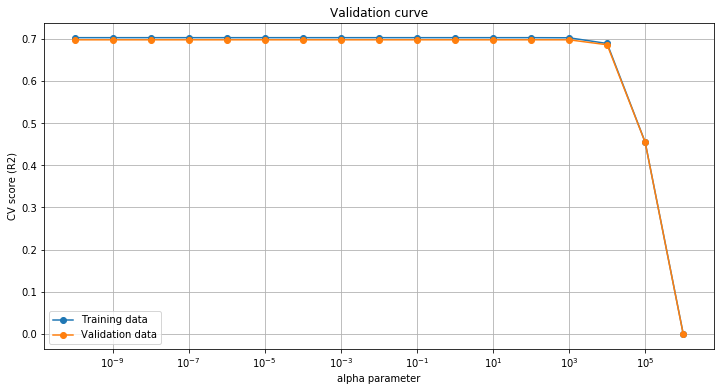### 2.3.3. SVR¶

Another alternative regression model is Support Vector Regression (SVR), which in this case proves much worse than the linear model:

In :
svr = SVR(kernel='linear', C=1)
eval_model(svr, X=X_std);

CV score: 0.088 ± 0.015

Out:
array([0.06641412, 0.07501845, 0.09492012, 0.09126587, 0.08698766,
0.07740102, 0.11327185, 0.07698808, 0.07872636, 0.1146053 ])

### 2.3.4. Random Forest Regressor¶

On the other hand, the Random Forest Regressor proves the most accurate model with an R2 score of 0.72, however this comes at the substantial computational cost. The random forest regressor takes over 400 times longer, although using the reduced feature set X_rfe, the computation takes less than half as long.

In :
forest = RandomForestRegressor(max_depth=4, random_state=0,
n_estimators=200)
eval_model(forest, X=X_std);

CV score: 0.721 ± 0.020

In :
%timeit eval_model(lr, X=X_std, out=False)

197 ms ± 42.4 ms per loop (mean ± std. dev. of 7 runs, 1 loop each)

In :
%timeit eval_model(forest, X=X_std, out=False)

1min 23s ± 7.3 s per loop (mean ± std. dev. of 7 runs, 1 loop each)

In :
%timeit eval_model(forest, X=X_rfe, out=False)

33.4 s ± 97.1 ms per loop (mean ± std. dev. of 7 runs, 1 loop each)


## 2.4. Feature Engineering¶

After having investigated options to eliminate irrelevant features above, I will now create additional features that improve the quality of the model.

Using sciki-learn's PolynomialFeatures(), I am expanding the feature matrix by quadratic and cubic terms. This improves the model quality to a score of 0.81 in case of polynomial order 2 and a standardized input matrix.

In :
# Second order (quadratic) polynomials with linear regression
pipe = Pipeline([('poly', PolynomialFeatures(2)),
('lr', lr)])

In :
eval_model(pipe, X=X_train); # quadratic terms, unstandardized features

CV score: 0.738 ± 0.039

In :
eval_model(pipe, X=X_std);   # quadratic terms, standardized features

CV score: 0.815 ± 0.019

In :
# Third order (cubic) polynomials with linear regression
pipe = Pipeline([('poly', PolynomialFeatures(3)),
('lr', lr)])

In :
eval_model(pipe, X=X_train); # cubic terms, unstandardized features


Training the model on a feature matrix containing cubic terms increases the training time substantially. Therefore, I will try to reduce the number of features in the following by using RFE to reduce the size of the feature matrix before adding cubic terms. However, this model does not yield useful predictions.

In :
pipe = Pipeline([('rfe', RFE(lr, 13, step=1)),
('poly', PolynomialFeatures(3)),
('lr', lr)])
eval_model(pipe, X=X_std);

CV score: -1.912 ± 8.104


### 2.4.3. Combine polynomial features with PCA¶

In this section, the size of the feature matrix will be reduced not through RFE before adding cubic terms, but using principal component analysis (PCA). First, I will train a linear regression model on the PCA-reduced feature matrix alone. Not surprisingly, this reduces the score:

In :
pipe = Pipeline([('pca', PCA(n_components=13)),
('lr', lr)])
eval_model(pipe, X=X_std);

CV score: 0.662 ± 0.023


Now, I'm adding cubic terms after reducing the feature matrix size. The combination of PCA and cubic terms yields a score of 0.77 which is significantly better than the score of the linear model alone.

In :
pipe = Pipeline([('pca', PCA(n_components=13)),
('poly', PolynomialFeatures(3)),
('lr', lr)])
eval_model(pipe, X=X_std);

CV score: 0.768 ± 0.074


In the following, I will test PCA with different values for n_components.

In :
scores_list = []  # List in which scores will be saved

# Loop over values for n_components
for n_components in range(7,17):

# Build pipe
pipe = Pipeline([('pca', PCA(n_components=n_components)),
('poly', PolynomialFeatures(3)),
('lr', lr)])

# Evaluate model, print, and save score in scores_list
cvs = eval_model(pipe, X=X_std, out=False);
print('n_components = %d :  %.3f pm %.3f' % \
(n_components, cvs.mean(), cvs.std()))
scores_list.append(cvs.mean())

n_components = 7 :  0.681 pm 0.042
n_components = 8 :  0.684 pm 0.041
n_components = 9 :  0.688 pm 0.042
n_components = 10 :  0.696 pm 0.046
n_components = 11 :  0.762 pm 0.037
n_components = 12 :  0.757 pm 0.049
n_components = 13 :  0.768 pm 0.074
n_components = 14 :  0.777 pm 0.035
n_components = 15 :  0.669 pm 0.108
n_components = 16 :  0.232 pm 0.379

In :
plt.figure(figsize=(12,6))
plt.xlabel('n_components')
plt.ylabel('CV score (R2)')
plt.plot(range(7,17), scores_list, marker='o')
plt.grid()
plt.show()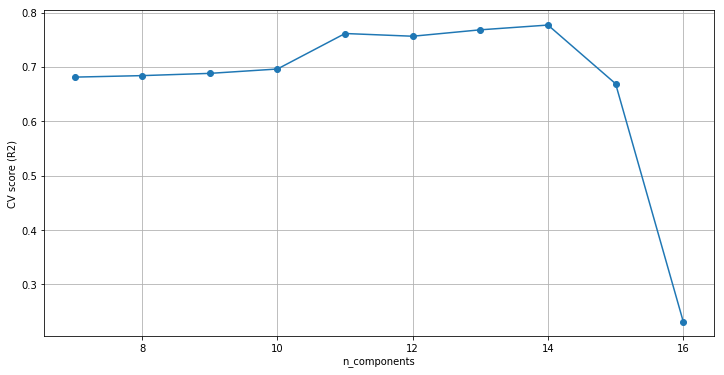As the above plot shows, the ideal number for n_components is 14, yielding a score of 0.78. Thus, the combination of PCA and adding cubic terms does not yield a better result than square terms alone.

## 2.5. Summary of Models¶

Different regressors: I started with a simple linear model (LinearRegression()), which achieved a score of 0.70. The related ridge and lasso models (Ridge(), Lasso()) which penalize large weights yield the same result, 0.70. A support vector classifier (SVR()) gave a score of only 0.09 but random forest regression (RandomForestRegressor()) gave a slightly better (the best) score with 0.72, but at significant computational cost.

Feature engineering: I then modified the feature set used by the model, and evaluated them with the linear model. First, I used polynomial features (PolynomialFeatures()) which improved the score to 0.74 but this result was improved even further to 0.82 when using scaled features. This is the overall best result.

Moving further to cubic terms reduced the score to 0.63, in addition the computational cost went up substantially. To combate that, I tried preprocessing the data with RFE (which yielded bad results) and PCA. After just reducing the dimensionality to 13 with PCA, the score was 0.66. But when PCA was used for preprocessing before creating cubic terms with PolynomialFeatures(3), the score went up to 0.78.

# 3. Visualization on a Map¶

The data includes geographic information in the form of latitude and longitude of the sold properties. In the following, I use Basemap to plot a fraction (10%) of the houses on a map, with their price colorcoded, blue being cheap and red being expensive.

In :
from mpl_toolkits.basemap import Basemap
from matplotlib.cm import bwr # import color map

plt.figure(figsize=(12, 12))

# Create map with basemap
m = Basemap(projection='cyl', resolution='i',
llcrnrlat = df['lat'].min()+0.1,
llcrnrlon = df['long'].min()-0.1,
urcrnrlat = df['lat'].max(),
urcrnrlon = df['long'].max()-0.6)

m.arcgisimage(service='ESRI_Imagery_World_2D',
xpixels=1500, verbose=True)

# Reducing number of properties shown
plot_df = df[::10]

for index, house in plot_df.iterrows(): # Loop over houses

# Get position on the map from geo coordinates
x,y = m(house['long'], house['lat'])

#  Get color from price
price_min = 200000
price_max = 800000
price_norm = (house['price'] - price_min) / (price_max - price_min)

rgb_exp = price_norm
rgb=[0,0,0]
for i in range(3): rgb[i] = int(bwr(rgb_exp)[i]*255)
color_hex = "#%02x%02x%02x" % (rgb, rgb, rgb)

#Plot data point
plt.plot(x,y, 'o', markersize=6, color=color_hex, alpha=0.6)

plt.show()

http://server.arcgisonline.com/ArcGIS/rest/services/ESRI_Imagery_World_2D/MapServer/export?bbox=-122.619,47.255900000000004,-121.91499999999999,47.77759999999999&bboxSR=4326&imageSR=4326&size=1500,1111&dpi=96&format=png32&f=image